## A diagonal aluminum alloy tension rod of diameter d and initial length l is used in a rectangularframe to prevent collapse. The rod can safe

Question

A diagonal aluminum alloy tension rod of diameter d and initial length l is used in a rectangularframe to prevent collapse. The rod can safely support a tensile stress of sallow. If d 5 0.5 in,l 5 8 ft, and sallow 5 20 kpsi, determine how much the rod must be stretched to develop thisallowable stress.

in progress 0
5 months 2021-08-24T21:57:04+00:00 1 Answers 6 views 0

The stretched length is 0.18816 in.

Explanation:

Given that,

Distance = 0.5 in

length = 8 ft

Tensile stress = 20 kpsi

We need to calculate the stretched length

Using formula of length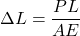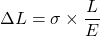Where,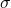= Tensile stress

L = length

E = young’s modulus of aluminum

Put the value into the formula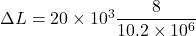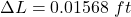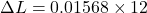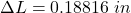Hence, The stretched length is 0.18816 in.